### Home > CALC > Chapter 2 > Lesson 2.1.1 > Problem2-14

2-14.
1. Given the function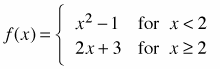, evaluate the following: Homework Help ✎

1. f(2)

2. As x → 2+, y → ?

3. As x → 2-, y → ?

4. What do the results from parts (b) and (c) indicate about the graph?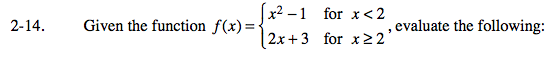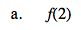Notice the inequality symbols. f(2) exists as part of the right side of f(x) not the left.

f(2) = 2(2)+3 = 7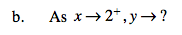Compare this question to part (a).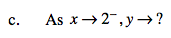Compare this question to part (a). Even though f(2) does not exist in the left side of the equation, since both sides approach x = 2 we can evaluate what it approaches.

x →2, y →(2)²−1 or y →3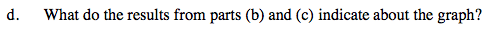Is f(x) continuous at x = 2? In other words, does f(x) approach the same y-value from the left and from the right of x = 2?